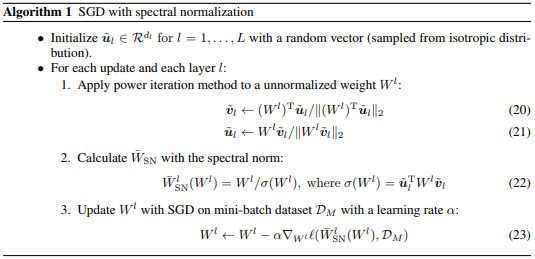## WHY?

The largest drawback of training Generative Adversarial Network (GAN) is its instability. Especially, the power of discriminator greatly affect the performance of GAN. This paper suggests to weaken the discriminator by restricting the functional space of it to stablize the training.

## Note

Matrix norm can be defined in various ways. One of them is p-norm, which can be defined as $\|A\|_p = sup_{x\neq 0}\frac{\|Ax\|_p}{\|x\|_p}$. This implies the largest changes in magnitude during linear transformation of A. The norm is called spectral norm when p = 2. Spectral norm of a matrix A can be seen as the largest singular value of matrix A. Also, this is equivalent to the square root of the largest eigenvalue of positive semi-definite matrix $A^*A$ ($A^*$ is conjugate transpose matrix of A).

## WHAT?

Spectral norm of A ($\sigma(A)$) can be defined as follows. $\sigma(A):=max_{h:h\neq 0}\frac{\|A\mathbb{h}\|_2}{\|\mathbb{h}\|_2} = max_{\|\mathbb{h}\|\leq 1}\|A\mathbb{h}\|_2\\$ Given a layer function g : $\mathbb{h}_{in} \rightarrow \mathbb{h}_{out}$, Lipschitz norm $\|g\|_{Lip}$ is eqal to $sup_{\mathbb{h}}\sigma(\nabla g(\mathbb{h}))$. If we assume g as linear layer ($g(\mathbb{h})=W\mathbb{h}$), then $\|g\|_{Lip} = sup_{\mathbb{h}}\sigma(\nabla g(\mathbb{h})) = sup_{\mathbb{h}}\sigma(W) = \sigma(W)$. Since most of Lipschitz norms of non-linear activation functions are bounded to 1, the Lipschitz norm of a discriminator can be bounded as follows. $\|f\|_{Lip} \leq \prod_{l=1}^{L+1}\sigma(W^l)\\$ Spectral normalization is normalizing the weights of a discriminator with their spectral norm so that the Lipschitz norm of the discriminator to be bounded by 1. $\bar{W}_{SN}(W) := \frac{W}{\sigma(W)}$ To approximate the spectral norm fast, we can use power iteration method. $\tilde{\mathbb{v}} \leftarrow \frac{W^T \tilde{\mathbb{u}}}{\|W^T\tilde{\mathbb{u}}\|_2}\\ \tilde{\mathbb{u}} \leftarrow \frac{W^T \tilde{\mathbb{v}}}{\|W^T\tilde{\mathbb{v}}\|_2}\\ \sigma(W)\approx \tilde{\mathbb{u}}^T W \tilde{\mathbb{v}}$If we analyze the gradient of spectrally normlized weights, we can see that the gradient is penalized when gradient of W is close to the first singular components, preventing gradient from too focusing on single direction.

## So?

SN showed better overall performance with various hyperparameter settings in CIFAR-10 and STL-10.

## Critic

I really need to review Linear Algebra.

Miyato, Takeru, et al. “Spectral normalization for generative adversarial networks.” arXiv preprint arXiv:1802.05957 (2018).